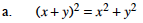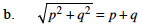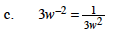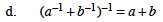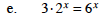### Home > PC > Chapter 1 > Lesson 1.2.2 > Problem1-84

1-84.
1.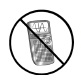Though many students think the following statements are true, each of them is actually FALSE. Confirm this by substituting simple numbers for the variables and doing the arithmetic. Note: Do not use 0 or 1; they are too special. Homework Help ✎

REMEMBER: EACH OF THESE STATEMENTS IS FALSE.

1. (x + y)2 = x2 + y2

2.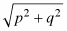= p + q

3. 3w2 =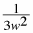4. (a1 + b1)−1 = a + b

5. 3 · 2x = 6 x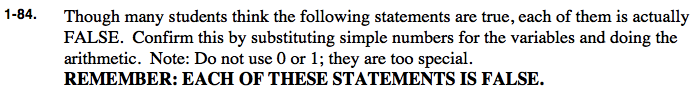Try substituting numbers such as 2, 3, 4, or 5.